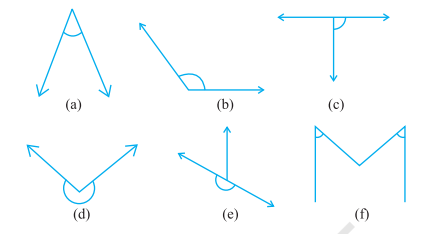### Chapter - 5 Understanding Elementary Shapes

Q
##### Understanding Elementary Shapes

Question:

1. Match the following :

(i) Straight angle        (a) Less than one-fourth of a revolution

(ii) Right angle           (b) More than half a revolution

(iii) Acute angle         (c) Half of a revolution

(iv) Obtuse angle      (d) One-fourth of a revolution

(v) Reflex angle        (e) Between 1/4 and 1/2 of a revolution

(f) One complete revolution

Solution:

(i) Straight angle        (c) Half of a revolution

(ii) Right angle           (d) One-fourth of a revolution

(iii) Acute angle         (a) Less than one-fourth of a revolution

(iv) Obtuse angle      (e) Between 1/4 and 1/2 of a revolution

(v) Reflex angle        (f) One complete revolution

2. Classify each one of the following angles as right, straight, acute, obtuse or reflex:Solution: (a) Acute angle, as it measures less than 90⁰.

(b) Obtuse angle, as it measures more than 90° but less than 180º.

(c) Right angle, as it measures 90º.

(d) Reflex angle, as it measures more than 180° but less than 360⁰.

(e) Sraight angle, as it measures 180º.

(f) Acute angle, as it measures less than 90⁰.